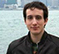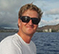# Deconvolution by least squares (Using the power of linear algebra in signal processing).

When we deal with our normal discrete signal processing operations, like FIR/IIR filtering, convolution, filter design, etc. we normally think of the signals as a constant stream of numbers that we put in a sequence, such as $x(n)$ with $n\in\mathbb{Z}$. This is at first the most intuitive way of thinking about it, because normally in a digital signal processing system (especially when applied in real time), we take some analogue signal from a sensor like a microphone, convert it to samples with an A/D converter and make use of each value for some calculation as they come along. But there is also another useful interpretation. We can choose an analysis window value $N$, and take $N$ consecutive samples of $x(n)$, to build a vector as:

$$\mathbf{x} = [x(0),x(1), \ldots, x(N-1)]^T$$

What this enables us to do, is to instead of thinking of a scalar signal $x(n)$, we now think of a vector signal

$$\mathbf{x}_k = [x(Nk), x(Nk+1), \ldots, x(Nk+N-1)]^T$$

To give an example of how we can take advantage of this approach, consider a simple FIR filter, with $M=4$ coefficients $h(n)$ with $n = 0,1,2,3$. Let $x(n)$ be the input and $y(n)$ be the output. And let's assume we are using a window size of $N=5$ If we use our vector interpretation and try to calculate directly all of the output samples that will result from the convolution of the first 5 samples of $x(n)$ with $h(n)$, we can put the operation in matrix form as follows

$$\mathbf{y}_k = \mathbf{H}\mathbf{x}_k$$

with

$$\mathbf{H} = \left[ \begin{array}{ccccc} h_{0} & 0 & 0 & 0 & 0 \\ h_{1} & h_{0} & 0 & 0 & 0 \\ h_{2} & h_{1} & h_{0} & 0 & 0 \\ h_{3} & h_{2} & h_{1} & h_{0} & 0 \\ 0 & h_{3} & h_{2} & h_{1} & h_{0} \\ 0 & 0 & h_{3} & h_{2} & h_{1} \\ 0 & 0 & 0 & h_{3} & h_{1} \\ 0 & 0 & 0 & 0 & h_{3} \\ \end{array}\right]$$

As it is expected, the length of the output sequence (number of samples different than zero in $y(n)$) is $N+M-1 = 5+4-1 = 8$. The structure of the matrix $\mathbf{H}$ is known as Toeplitz (or constant diagonal).

So, it seems that we haven't achieved anything useful besides expressing our operation in a fancier way with a matrix. But, now imagine we know the output of the filter and have the impulse response, and want to recover the input. This is known as deconvolution. In the traditional style of working with the filters, you would probably compute the Z-transform of the impulse response, invert it and obtain the Z-transform of the inverse filter, then anti transform and obtain an infinite impulse response that you probably have to implement with a feedback structure. But, here is where linear algebra comes to the rescue. By examining the from of the matrix $\mathbf{H}$ we can easily see that it is full rank. I will not prove it in a mathematically rigorous way, but just think of this: If you want to express the first column as linear combination of any of the other ones, because the first element is $h_0$ (non zero by hypothesis, otherwise our impulse response length $M$ could be one shorter) we can't because they all have a zero component as the first column element. And you can follow the same argument for the rest of the columns. So, we can find the value of the vector $\mathbf{x}_k$ by using the famous Least squares formula

$$\mathbf{x}_k = \left(\mathbf{H}^{T}\mathbf{H}\right)^{-1}\mathbf{H}^{T}\mathbf{y}_k$$

And this result holds exactly, it will obtain precisely the same samples that were put at the input. Now you may ask yourself: Wait a minute, you said a moment ago that to apply the inverse filter we would need an IIR filter which is recursive, and multiplying the vector $\mathbf{y}_k$ by this matrix does not do any recursion, how can this be possible?. True. But it is also not a FIR filter, because the matrix $\left(\mathbf{H}^{T}\mathbf{H}\right)^{-1}\mathbf{H}^{T}$ does not have the same Toeplitz structure as $\mathbf{H}$, the rows will probably not be all a shifted version of the previous one. In a sense, it might be like applying a time-varying FIR filter, because to obtain the first sample of $x(n)$ you multiply by some coefficients and add everything, but for the second sample, you do not shift the coefficients and do the same, you multiply by completely different numbers.

So as we can see, thinking your processing systems in terms of matrix operations can yield some interesting results and some methods that are not so obvious if you think in the "traditional" way.

[ - ]
Comment by November 22, 2015What do you mean by minimum phase signal? I know a system can have the property of being minimum phase. Do you apply the same term to a signal thinking the samples as an impulse response of "some" system? Irregardless of that, I think you are partially right, this result will not "always" be true, because i cheated with the numbers so that it is correct. But if the convolution matrix is skinny (more rows than columns), then it is guaranteed to be full rank (something i did not prove but suggested it might be easy to see), so the pseudo inverse will always exist, an applying it to $y_k$ will return exactly the input $x_k$ independently of any delay, or where you put the zero of your time axis. In practice, of course, when the length of the sequence is too long, it becomes impractical to create a huge matrix to do the inverse all at once, and we would have to partition in many blocks, but that's for another post :)
[ - ]
Comment by November 21, 2015Augustin - Nice post!
I think the result holds for minimum phase signals, for non-minimum phase signals you need to add a processing delay - then, the MoorePenrose pseudoinverse formula is referred to as the spiking filter (I think Robinson termed it this way). I wanted to give you a better reference than my own, but couldn't find one on the fly: Partial dereverberation used to characterize open circuit scuba diver signatures.
~kai

Partial dereverberation used to characterize open circuit scuba diver signatures

To post reply to a comment, click on the 'reply' button attached to each comment. To post a new comment (not a reply to a comment) check out the 'Write a Comment' tab at the top of the comments.